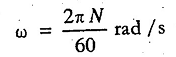### Angular Velocity

What is Angular Velocity? Give Definition and Formula?

It is the rate of change of angular displacement of a body. It is usually expressed in revolutions per minute (r.p.m.) or radians per second (rad/s). It is denoted by a Greek letter ω (Omega). Since it has magnitude and direction, therefore it is a Vector quantity.

If a body is rotating at N r.p.m, then corresponding angular velocity,Note: If the body is rotating at ω rad/s along a circular path of radius r, then its linear velocity (v) is given by:

v = ω.r